## Source CodeInpainting Network, 1.0 (Feb, 2018)
Globally and locally consistent image completion network
This code is the implementation of the "Globally and Locally Consistent Image Completion" paper. It contains the pre-trained model and example usage code.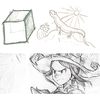Sketch Simplification Network, 1.0 (Dec, 2017)
Sketch Simplification Convolutional Neural Network
This code is the implementation of the "Learning to Simplify: Fully Convolutional Networks for Rough Sketch Cleanup" and "Mastering Sketching: Adversarial Augmentation for Structured Prediction" papers. It contains pre-trained models and example usage code.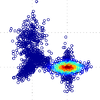GFMM, 2.0 (Jul, 2016)
Geodesic Finite Mixture Models
This code is an implementation of the Geodesic Finite Mixture Models written in matlab. The core of the algorithm consists of a single file which can be called to perform the clustering. Additionally, several examples are provided to generate the figures from the paper.StyleNet, 1.0 (Jun, 2016)
Fashion style in 128 floats
This code is the implementation of the "Fashion Style in 128 Floats: Joint Ranking and Classification using Weak Data for Feature Extraction". It contains the best performing feature extraction model explained in the paper.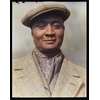Colorization Network, 1.0 (Apr, 2016)
Let there be Color! Colorization Network
This code is the implementation of the "Let there be Color!: Joint End-to-end Learning of Global and Local Image Priors for Automatic Image Colorization with Simultaneous Classification" paper. It contains the pre-trained model and example usage code.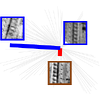Deep Descriptor, 1.0 (Feb, 2016)
Deep Convolutional Feature Point Descriptors
This code is the implementation of the "Discriminative Learning of Deep Convolutional Feature Point Descriptors" paper. It contains the pre-trained models and example usage code.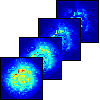DaLI, 1.0 (Jan, 2015)
Deformation and Light Invariant feature point descriptor
This is an implementation of the Deformation and Light Invariant (DaLI) descriptor. The core of the library is written in C. Additionally a Matlab/Octave interface is provided.Clothes Parsing, 1.0 (Dec, 2014)
Clothes Parsing
This code is the implementation of the "A High Performance CRF Model for Clothes Parsing" paper. It contains all the code for being able to learn and run inference. The features for the Fashionista dataset must be downloaded separately.libdq, 2.2 (Feb, 2013)
Library for using and manipulating unit dual quaternions to describe rigid body motions
This is a library for using and manipulating unit dual quaternions (Clifford algebra Cl^+(0,3,1). Unit dual quaternions are useful for describing rigid body movements using screw theory. Main applications of using unit dual quaternions are found in kinematics. The core of the library is written in C. However, a Lua interface is also provided which is installable by means of LuaRocks. This provides a simple interface for the quick prototyping of projects.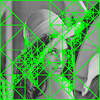bttc, 1.0 (Mar, 2012)
Small library to handle B-Tree Triangular Coding (BTTC)
This library calculates the faces obtained by B-Tree Triangular Coding (BTTC). This is usually for subdividing an image into a triangular mesh. The core library is written in C but an octave/matlab interface is provided. The main focus of this library is simplicity. The code is simple enough to directly integrate it into another program as it is a single C code file with no external dependencies.ArtTreeKS, 1.0 (Mar, 2012)
Kinematic synthesis solver for tree topologies
ArtTreeKS is a dedicated kinematic synthesis solver for tree topologies. It is designed specifically for finite position kinematic synthesis and has support for both position constraints as well as velocity or acceleration constraints. The solver employed is a hybrid solved that is based on a Genetic Algorithm (GA) built on top of a Levenberg-Marquadt local optimizer. This allows obtaining solutions for very complex tree topologies.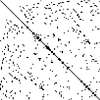ceigs, 1.1 (Jan, 2012)
C Wrapper for the ARPACK (Arnoldi Iteration) Library
This is a simple C frontend for ARPACK. This allows easy access to calculating a subset of eigenvectors and eigenvalues of sparse matrices. Specifically it can solve two problems: - Av = vd - Av = Mvd Where A, M are sparse matrices, v is the subset of eigenvectors and d is the diagonal matrix of eigenvalues.